# Boolean operations in solid modelingThere is a technique for obtaining complex volumes from the addition or subtraction of other simpler volumes called “primitive”; such Solid operations known as “Boolean operations”.

## PrimitivesThe various modeling (3D edition) used to create animated scenes and characters, include among their tools the ability to create objects from elemental solids and surfaces.

Some geometric elements underlying the model described: sphere, plane, cylinder, cone, bull …

These basics are called “graphics primitives” and by operators come together to build more complex elements as discussed later.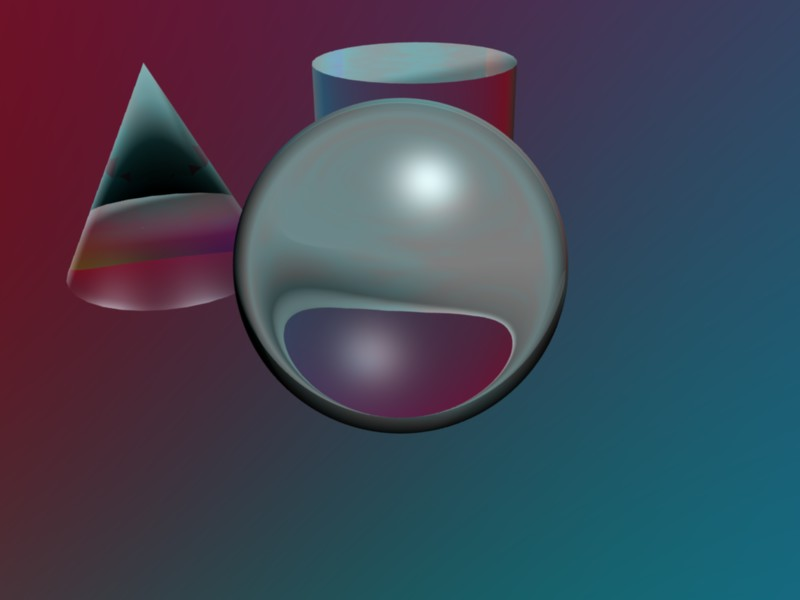The primitives are defined with unit sizes and the origin.

We see the surface of an object and not inside; if we use Boolean operations we think about the volume and differentiate between the surface or “border” the object and the volume that it occupies.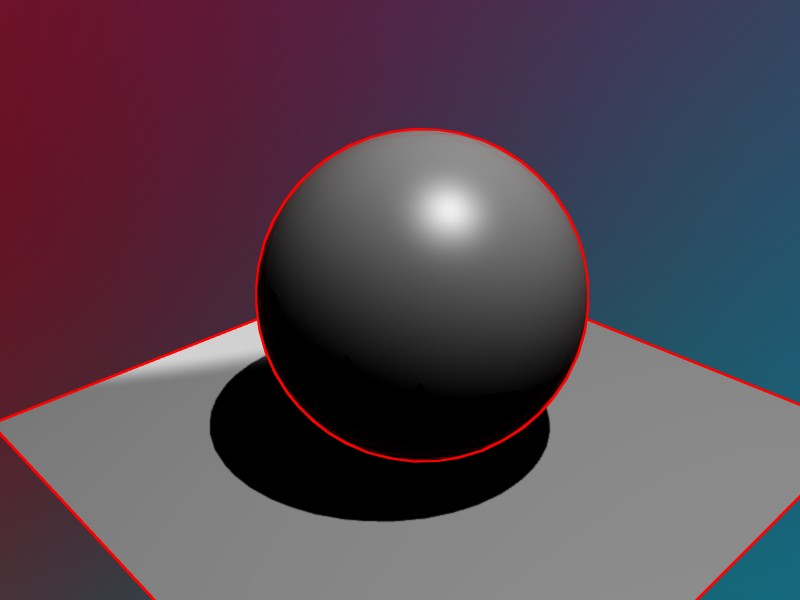For example, in the plane border will be determined by the edges or corners of the polygon that represents it as the plane does not have volume. Sin embargo, in a sphere, the border of the object is its surface, going to call “outline” curve that holds when we represent an image.

### Plans, surfaces and volumes

The simplest primitive is the triangle. From this geometric element surfaces are generated with different degrees of complexity. For example, with the triangles can form a rectangle plan, several that share a polygon center (that simulates the one closest circle), etc..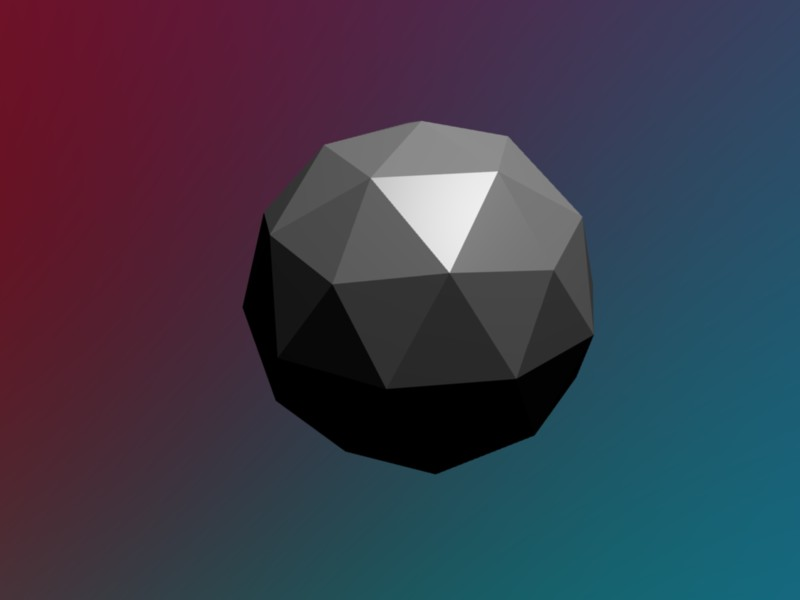If we build a surface formed by triangles that share vertices and we can consider the volume close as what's on “inside” border that forms the surface.

A sphere can be approximated by a polyhedral shape, and by rendering techniques (interpolations) obtain images with shapes smoothed.The geometric model can give rise to different representations. changing the perception of the object.

In the second display, the polyhedron adopts a sphere appearance thanks to the display model, however, the outline of the shape is the same.

This degrades polygonal contour perception spherical. To correct this you need to increase the number of faces of the polyhedron.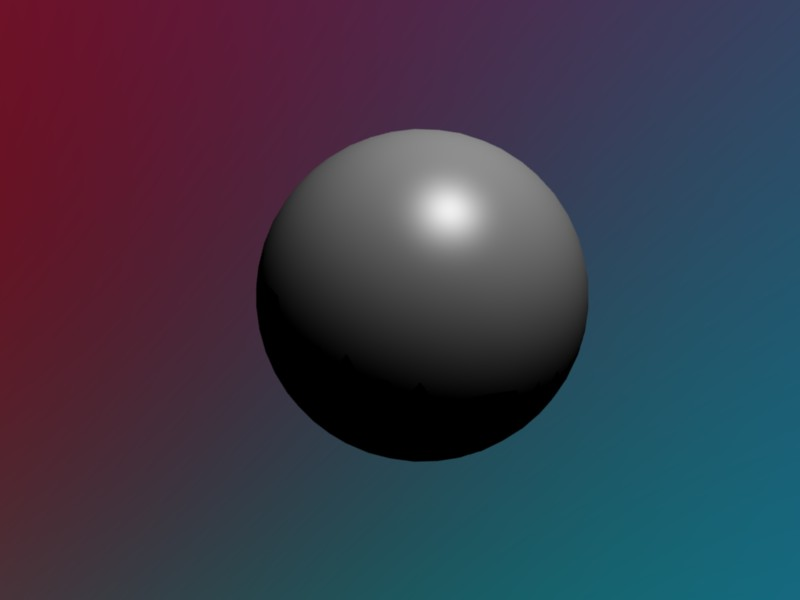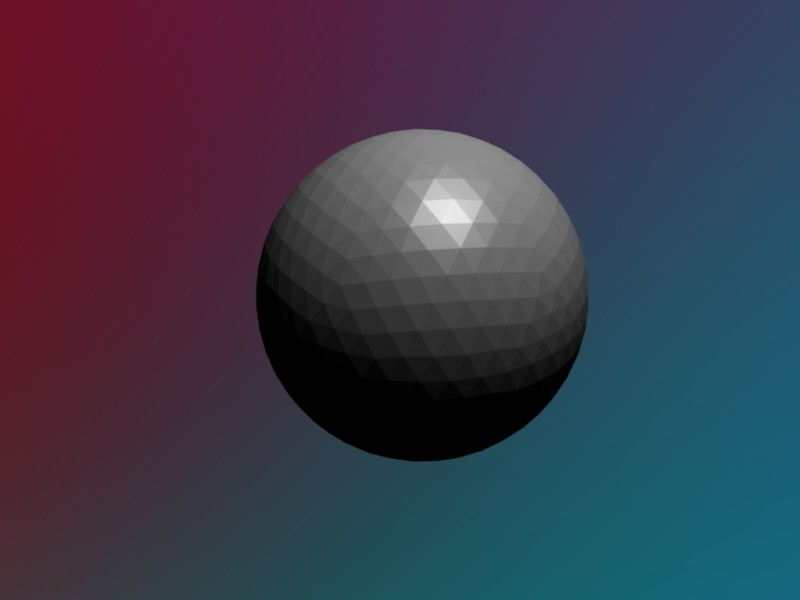The new approach to improving visualization spherical surface at the expense of increasing the volume of surfaces included in the calculations of the picture. The volume of the sphere also closely approximates, allowing more detailed editing model.

## Boolean operations

Objects as the sphere, cylinder, cone etc., may be defined analogously, called basic volumes obtained as stated “graphics primitives”.

The volume of these objects can be characterized by a simple law, for example:

A point in space is inside a volume if there is no line through the point and do not cut the border (surface). If there is at least one, this is exterior.

Boolean operations are based on models that are discussed in Boolean algebra. The concepts of addition, remains, common part etc. are employed in this technique solids modeling.

### Sum

By adding two volumes, the total volume is equal to or less than the sum of their volumes, since there is a common part between the elements.We add for example a sphere and a cube:

the resulting object will occupy, at the most, the space occupied by two elements;

interior points to one of them will therefore object points resulting from the sum.

Distinguish between different volumes or regions of space in this example:

• Outside: Points that are not interior to any object.
• Interior: Points that are interior to one or both objects.

The new object boundary sum, uses parts of the boundaries of the primitives as seen in the image.

### IntersectionThe intersection of two objects is also known in some applications as “common part”.

Is formed by internal elements which are the two objects used in the operation.

Again borders are formed by parts of the two boundaries involved.

The intersection is commutative and that the body is the same result of the intersection between A and B than that formed by the intersection between B and A

### Difference

The two previous operations are performed regardless of the order in which the elements are defined.Sin embargo the difference is not has this property commutative. Not the same take the volume to the body B, to remove B to A.

Boolean operation “B – A”, namely, obtaining the difference between B and A, is equivalent to saying “deprive the common B between B and A”.

If we remove the sphere cube, get a smaller volume, but greater than the difference in volumes, and that parts of the area that are not in contact with the hub can not be subtracted.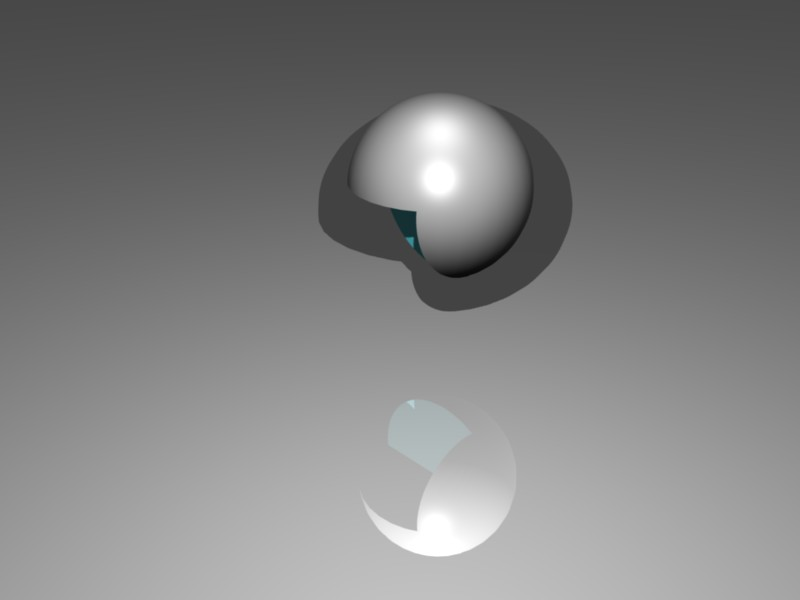The difference between subtracting one element to another, or reverse the order of objects in the operation is markedly different.

Normally indicate the object which endures and then subtract, although it may depend on the implementation in each program.

If you want to see an example of construction using this technique, sight how to generate a nut with Boolean operations.